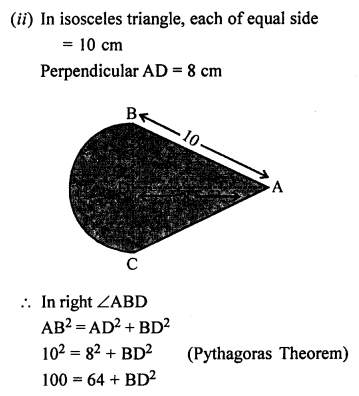## ML Aggarwal Class 7 Solutions for ICSE Maths Chapter 16 Perimeter and Area Ex 16.3

Take π = $$\frac { 22 }{ 7 }$$, unless stated otherwise.
Question 1.
Find the circumference of the circles with the following radius:
(i) 7 cm
(ii) 21 cm
(iii) 28 mm
(iv) 3.5 cm
Solution:
(i) Radius of the circle (r) = 7 cm
Circumference = 2πr = 2 × $$\frac { 22 }{ 7 }$$ × 7 = 44 cm
(ii) Radius of the circle (r) = 21 cm
Circumference = 2πr = 2 × $$\frac { 22 }{ 7 }$$ × 21 = 132 cm
(iii) Radius of the circle (r) = 28 mm
Circumference = 2πr = 2 × $$\frac { 22 }{ 7 }$$ × 28 mm = 176 mm
(iv) Radius of the circle (r) = 3.5 cm
Circumference = 2πr = 2 × $$\frac { 22 }{ 7 }$$ × 3.5 cm = 22 cm

Question 2.
Find the area of the circles, given that:
(ii) diameter = 49 m
(iii) diameter = 9.8 m
Solution:
(i) Radius (r) = 14 mm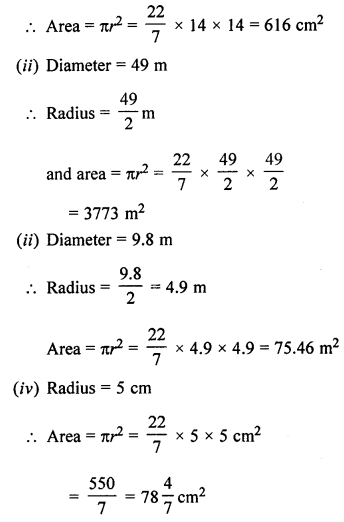Question 3.
Find the circumference and area of a circle of radius 20 cm. (use π = 3.14)
Solution:
Radius of a circle (r) = 20 cm
Circumference = 2πr = 2 × 3.14 × 20 cm = 125.60 = 125.6 cm
and area = πr2 = 3.14 × 20 × 20 cm2 = 1256 cm2

Question 4.
The minute hand of a tower clock is 1.4 m long. How far does the tip of the hand move in 1 hour?
Solution:
Length of minute hand (r) = 1.4 m
Circumference = 2πr = 2 × $$\frac { 22 }{ 7 }$$ × 1.4 m = 8.8 m
Distance covered = 8.8 m

Question 5.
A gardener wants to fence a circular garden of diameter 21 m. Find the length of the rope he needs to purchase if he makes 2 rounds of the fence. Also find the cost of the rope, if it costs ₹ 4 per metre.
Solution:
Diameter of a circular garden = 21 m
Circumference = πd= $$\frac { 22 }{ 7 }$$ × 21 = 66 m
Length of rope for 2 rounds of fence = 66 × 2 = 132 m
Rate of rope = ₹ 4 per metre
Total cost = 132 × 4 = ₹ 528

Question 6.
If the circumference of a circle exceeds its diameter by 30 cm, find the radius of the circle.
Solution:
Let fadius of a circle = r
Then diameter = 2r
and circumference = 2πr
According to the condition,
2πr – 2r = 30
r(2π – 2) = 30Question 7.
Find the length of the diameter of a circle whose circumference is 44 cm.
Solution:
Circumference of a circle = 44 cm
Circumference of circles = 2πr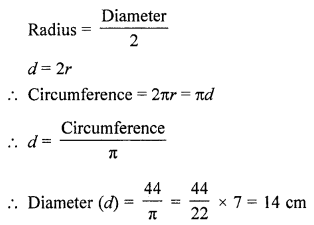Question 8.
The circumference of a circle is 31.4 cm. Find the radius and the area of the circle. (Take π = 3.14)
Solution:
Circumference of a circle = 31.4 cm
Circumference = 2πrand area = πr2 = 3.14 × 5 × 5 = 78.5 cm2

Question 9.
Find the radius and the circumference of a circle whose area is 144π cm2.
Solution:
Area of a circle = 144π cm2
Area = πr2and circumferene = 2πr = 2 × π × 12 = 24π cm

Question 10.
How many times will the wheel of a car rotate in a journey of 88 km, given that the diameter of the wheel is 56 cm?
Solution:
Total distance covered = 88 km = 88 km × 1000 m = 88000 m
Diameter of a wheel (d) = 56 cm
Circumference = 2πr = πd = $$\frac { 22 }{ 7 }$$ × 56 = 176 cm
Number of rotation of the wheelQuestion 11.
From square cardboard of side 21 cm, a circle of the maximum area is cut out. Find the area of the cardboard left.
Solution:
Side of a square cardboard = 21 cm
A circle of the maximum area is cut out from is
Diameter of circle = 21 cm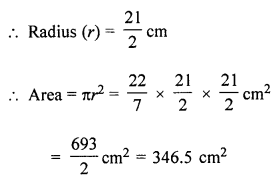Total area of the cardboard = 21 × 21 cm2 = 441 cm2
Area of remaining cardboard = 441 – 346.5 = 94.5 cm2

Question 12.
A piece of wire is bent in the shape of an equilateral triangle of side 4.4 cm. If this wire is rebent to form of a circle, find the radius and the area of the circle.
Solution:
Side of equilateral triangular shaped wire = 44 cm
Perimeter = 4.4 × 3 cm = 13.2 cm
After rebenting it into a circular shape the circumference = 13.2 cm
Radius = $$\frac { Circumference }{ 2\pi }$$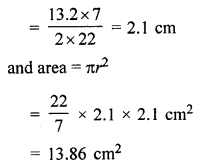Question 13.
A wire is in the form of a square of side 27.5 cm. It is straightened and bent into the shape of a circle. Find the area of the circle.
Solution:
Side of square shaped wire = 27.5 cm
Perimeter = 27.5 × 4 = 110 cm
Now after rebenting it into a circle the circumference = 110 cmQuestion 14.
A wire is in the form of a rectangle 18.7 cm long and 14.3 cm wide. If this wire is reshaped and bent in the form of the circle, find the radius and the area of the circle so formed.
Solution:
Length of rectangular wire (l) = 18.7 cm
and breadth (b) = 14.3 cm
Perimeter = 2(l + b) = 2(18.7 + 14.3) cm = 2 × 33 = 66 cm
After rebenting it into a circle circumference = 66 cm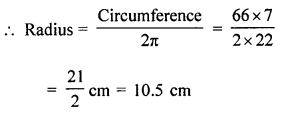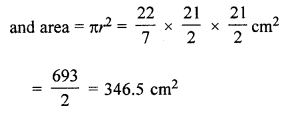Question 15.
The diameter of a circular park is 84 m. On its outside, there a 3.5 m wide road. Find the cost of constructing the road at ₹ 240 per m2.
Solution:
Diameter of a circular park = 84 m
Inner radius (r) = $$\frac { 84 }{ 2 }$$ = 42 m
and outer radius (R) = 42 + 3.5 = 45.5 m
Area of road = π(R2 – r2)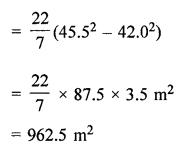Rate of constructing the road = ₹ 240 per m2
Total cost = ₹ 962.5 × 240 = ₹ 231000

Question 16.
A circular pond is surrounded by a 2 m wide circular path. If the outer circumference of the circular path is 44 m, find the inner circumference of the circular path. Also, find the area of the path.
Solution:
The outer circumference of circular path = 44 m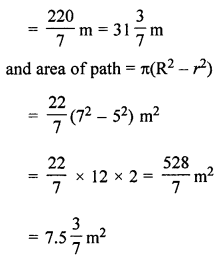Question 17.
In the given figure, the area enclosed between the concentric circles is 770 cm2. If the radius of the outer circle is 21 cm, calculate the radius of the inner circle.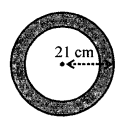Solution:
Area enclosed by two concentric circles = 770 cm2
Radius of outer circle (R) = 21 cmQuestion 18.
From a circular card sheet of radius 14 cm, two circles of radius 3.5 cm and a rectangle of length 3 cm and breadth 1 cm are removed (as shown in the given figure). Find the area of the remaining sheet.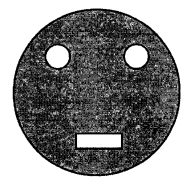Solution:
Radius of circular card sheet (R) = 14 cm
Radius of smaller circles = 3.5 cm
and length of rectangle = 3 cm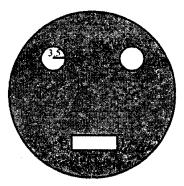Now area of bigger circular sheet = πR2
= $$\frac { 22 }{ 7 }$$ × 14 × 14 = 616 cm2
Area of 2 smaller circles = 2 x πr2
= 2 × $$\frac { 22 }{ 7 }$$ × 3.5 × 3.5 cm = 77 cm2
and area of rectangle = 3 × 1 = 3 cm
Area of shaded portion = 616 – (77 + 3) cm2 = 616 – 80 = 536 cm2

Question 19.
Calculate the length of the boundary and the area of the shaded region in the following diagrams. All measurements are in centimetres.
(i) The unshaded part is a semicircle.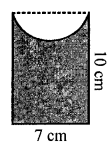(ii) Four semicircles on a square.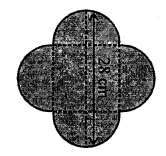Solution:
(i) Length of rectangle = 10 cm
Area = l × b = 10 × 7 = 70 cm2
Radius of semicircle on one side = $$\frac { 7 }{ 2 }$$ cm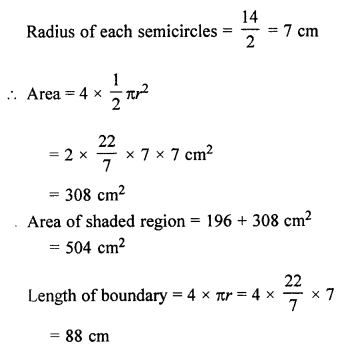Question 20.
Find the perimeter and the area of the shaded region in the following figures. All measurements are in centimetres.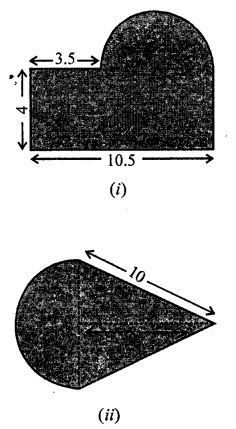Solution:
(i) Length of rectangular part = 10.5 cm